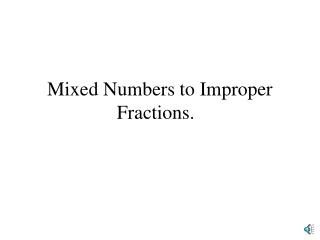DownloadDownload PresentationMixed Numbers to Improper Fractions.

# Mixed Numbers to Improper Fractions.

Télécharger la présentation## Mixed Numbers to Improper Fractions.

- - - - - - - - - - - - - - - - - - - - - - - - - - - E N D - - - - - - - - - - - - - - - - - - - - - - - - - - -
##### Presentation Transcript

1. Mixed Numbers to Improper Fractions.

2. Lets say you have a mixed number of 1 and 5/8 You can change this into the number 13/8. For converting mixed numbers to improper fractions, the first thing you do is multiply. For example, the mixed number 3 and 4/5 you multiply the whole number by the denominator. 3x5= 15

3. Then you add the numerator to your answer 15 + 4 which is 19. So the 19 goes on the top and then the denominator from the mixed fraction goes on the bottom so you end up with the improper fraction 19/5.

4. Changing Improper Fractions to Mixed Numbers. • The first step to changing improper fractions to mixed numbers is to divide. For example, the fraction 16/3 can be changed. The first step is to divide. You divide the 3 into 16 and you get 5 with a remainder of 1.

5. 5 16 3 Your remainder is one. So the remainder will go in the numerator: - 15 1 1 5 So far your mixed number should look like this.

6. The denominator from the improper fraction goes on the denominator of the mixed number: 1 5 3 16 3 The number 5 is the whole number of the fraction, and the remainder of what you had is the numerator. Then the denominator stays the same. So your answer would look like this: This is how you change improper fractions to mixed numbers. 1 5 3